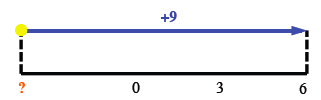### Home > MC2 > Chapter 2 > Lesson 2.1.3 > Problem2-41

2-41.

Solve the number puzzles below. 2-41 HW eTool1. If I add $9$ to my number, I get $6$. What is my number?Based on the number line, what was the original number?

2. If I start at $-5$ on a number line and end up at $-8$, what direction did I move? How many units did I move?

Experiment with the number line on the eTool below and use your observations to answer the question.

3. If I moved up $8$ and then moved down $8$, what can you tell me about my ending position?

• Try making your own number line (this one should be vertical).
Draw arrows $8$ up and then $8$ down and see where you land.

It is the same as the starting position. Can you see why?

Click the link at right for the full version of the eTool: 2-41 HW eTool